I need support with this Calculus question so I can learn better.

1.

Write and then solve for the differential equation for the statement: “The rate of change of y with respect to x is inversely proportional to y2.” (10 points)

2.

Solve the differential equation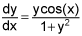with the initial condition y(0) = 1. (10 points)

3.

a. Solve the differential equation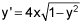b. Explain why the initial value problemwith y(0) = 4 does not have a solution.

4.

The table below gives selected values for the function f(x). Use a trapezoidal estimation, with 6 trapezoids to approximate the value of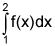. Give 3 decimal places for your answer. (10 points)

x 1 1.1 1.2 1.5 1.7 1.9 2.0
f(x) 1 2 4 6 7 9 10

5.

Using 4 equal-width intervals, show that the trapezoidal rule is the average of the upper and lower sum estimates for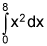. (10 points)

1.

The figure below shows the graph of f ‘, the derivative of the function f, on the closed interval from x = -2 to x = 6. The graph of the derivative has horizontal tangent lines at x = 2 and x = 4.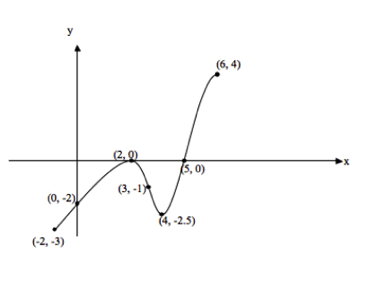Find the x-value where f attains its absolute maximum value on the closed interval from x = -2 to x = 6. Justify your answer. (10 points)

2.

A car travels along a straight road for 30 seconds starting at time t = 0. Its acceleration in ft/sec2 is given by the linear graph below for the time interval [0, 30]. At t = 0, the velocity of the car is 0 and its position is 10.

What is the velocity of the car when t = 6? You must show your work and include units in your answer.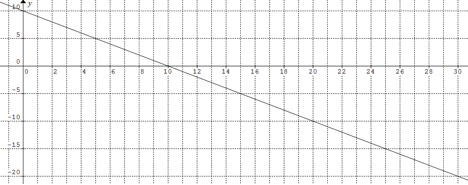(10 points)

3.

Show that f(x) = 2000x4 and g(x) = 200x4 grow at the same rate. (10 points)

4.

A radar gun was used to record the speed of a runner (in meters per second) during selected times in the first 2 seconds of a race. Use a trapezoidal sum with 4 intervals to estimate the distance the runner covered during those 2 seconds. Give a 2 decimal place answer and include units. (10 points)

t 0 0.5 1.2 1.5 2
v(t) 0 4.5 7.8 8.3 9.0

5.

Water flows into a tank according to the rate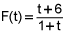, and at the same time empties out at the rate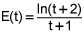, with both F(t) and E(t) measured in gallons per minute. How much water, to the nearest gallon, is in the tank at time t = 10 minutes. You must show your setup but can use your calculator for all evaluations. (10 points)